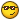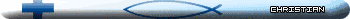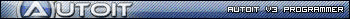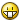## Recommended Posts

I have seen many examples on the internet about reflecting a point across the x or y axis but I need to reflect a point across any line. Let's say I have point (x, y) and I want to reflect it across a line. I have two points on the line: (a, b ) and (c, d). What would the two equations be to find the x and y values of the resulting point? Thanks.

Edited by erifash

##### Share on other sites

Only way I can think of is awfully trial-and-error, but the concept might help: (x,y)' (the reflected point...read the apostrophe as 'prime') is the other point at which the distance between (x,y) & (a,is the same as the distance between (x,y)' & (a,AND that the distance between (x,y) & (c,d) is the same as the distance between (x,y)' & (c,d).

The formula for finding the distance between (x,y) and (a,is:

`\$dist=sqrt((x-a)^2+(y-b)^2)`

So to find your (x,y)', you'll need to check "all" points for one that satisfies that condition - so to start, store \$distance1 as the distance between (x,y) and (a,, then store \$distance2 as the distance between (x,y) and (c,d). Now start checking points to find another one that is \$distance1 from (a,AND \$distance2 from (c,d).

Now, I'm sure an actual mathematician can tell you how better to find the approx. area around which to check for your (x,y)', but I can't think of it.

The other thing I just thought of is you might store into an array a thousand or so coordinates which are \$distance1 from (a,then compare only those coordinates to find the one that's closest to \$distance2 from (c,d). That will remove some of the endless searching of all possible points ;-)"There are 10 types of people in this world - those who can read binary, and those who can't.""We've heard that a million monkeys at a million keyboards could produce the complete works of Shakespeare; now, thanks to the Internet, we know that is not true." ~Robert Wilensky0101101 1001010 1100001 1101101 1100101 1110011 0110011 1001101 10001110000101 0000111 0001000 0001110 0001101 0010010 1010110 0100001 1101110

##### Share on other sites

...! Yeah, I had figured out the algorithm a while ago but I've lost it. I know it can be done just by using two equations. I also tried searching around some more and found this website but I can't understand it that well. It looks useful, can anyone explain it to me?EDIT: Here is a quick drawing of what I am trying to accomplish.

EDIT2: Here is another link http://www.topcoder.com/tc?module=Static&a...try2#reflection

Edited by erifash

##### Share on other sites

...! Yeah, I had figured out the algorithm a while ago but I've lost it. I know it can be done just by using two equations. I also tried searching around some more and found this website but I can't understand it that well. It looks useful, can anyone explain it to me?EDIT: Here is a quick drawing of what I am trying to accomplish.

EDIT2: Here is another link http://www.topcoder.com/tc?module=Static&a...try2#reflection

I don't think trial-and-error testing of points is required. I think you can get the reflection of coordinates x,y (call them x',y') by direct calculation. One thing you haven't defined that will be required: How will you define the line of reflection? You have choices:

1. Give a point (a,and the slope of the line through that point (m) as a number

2. Give A and B where y = Ax + B

3. Give A and B where x = Ay + B

4. Give A, B, and C where Ax + By + C = 0

5. Etc., more than my non-mathmatical mind can come up with right now...

6. Give two points on the line (a,b and c,d) (added after comment below)

What I'm saying is if you clearly define how you will input the definition of the line of reflection into the function, I think we can write a function that will take x,y and give you x',y' by direct calculation.

As an example, if you chose option 1 above:

```; Function _Reflect()
; Takes as input a point (\$a, \$b) and slope (\$m) to define a line of reflection,
;    and a point (\$x, \$y) to reflect across that line.
; Returns an array of the reflected coordinates,
;    where \$Array = x', and \$Array = y'
Func _Reflect(\$a, \$b, \$m, \$x, \$y)
Local \$aRet

; Do the math voodoo here...

Return \$aRet
EndFunc```

The math voodoo won't be that hard once you figure out what you want the inputs to the function to be.Edit: Had to turn off smilies to stop the buggers popping up in my text!

Edited by PsaltyDS

Valuater's AutoIt 1-2-3, Class... Is now in Session!For those who want somebody to write the script for them: RentACoder"Any technology distinguishable from magic is insufficiently advanced." -- Geek's corollary to Clarke's law

##### Share on other sites

Okay, I guess I'm not being clear here. Here is what I want:

Given two points on a line (a, b ) and (c, d) reflect point (x, y) across the line.

Here is some code I already have started:

```;Reflects point (\$x,\$y) over line segment (\$a,\$b)(\$c,\$d)
Func _Reflection( \$x, \$y, \$a, \$b, \$c, \$d )
Local \$ret
\$ret = 0;x coordinate
\$ret = 0;y coordinate
Return \$ret
EndFunc```

Of course, it doesn't work. Can anyone here perform some "math voodoo" to make it work?##### Share on other sites

Okay, I guess I'm not being clear here. Here is what I want:

Given two points on a line (a, b ) and (c, d) reflect point (x, y) across the line.

Here is some code I already have started:

Of course, it doesn't work. Can anyone here perform some "math voodoo" to make it work?well... why not just make a triangle find the height, and subtract double height for each coordinate

```;Reflects point (\$x,\$y) over line segment (\$a,\$b)(\$c,\$d)
Func _Reflection( \$x, \$y, \$a, \$b, \$c, \$d )
\$dist1 =sqrt((\$x-\$a)^2+(\$y-\$b)^2); from x,y to a,b
\$dist2 =sqrt((\$x-\$c)^2+(\$y-\$d)^2); x,y to c,d
\$dist3 =sqrt((\$a-\$c)^2+(\$b-\$d)^2); a,b to c,d
;*********find the area
\$sp=(\$dist1+\$dist2+\$dist3) /2
\$area = sqrt(\$sp*(\$sp-\$dist1)*(\$sp-\$dist2)*(\$sp-\$dist3))
;**********solve for height
\$height = 2*\$area / \$dist3
If \$b > \$d then; line goes " \"
;point above or below? <------------------------ help on concluding this
\$x1 = \$x-(\$height*2)
\$y1 = \$y-(\$height*2)
Elseif \$d > \$b then ; line is " / "
\$x1 = \$x+(\$height*2)
\$y1 = \$y-(\$height*2)
Else ;line is horizontal
If \$y >\$c then
\$x1 = \$x
\$y1 = \$y-(\$height*2)
Elseif \$y>\$c then
\$x1 = \$x
\$y1 = \$y+(\$height*2)
Else
SetError(1);this means coord was on horizontal line
Endif
Endif
Local \$ret
\$ret = \$x1;x coordinate
\$ret = \$y1;y coordinate
Return \$ret
EndFunc```

I think thats the "math voodoo" you want, its onl partially done,

so it wont work for vertical lines (horizontal is done though)

and only works for diagonals if layout is:

(Point) line like "/"

or

line like "\" (point)

I'll finish tommorrow

What does this have to do with pong anyway?

GRRRRR this is too dificult

can someone help me find which side of a diagonal line the point is on?

Edited by Paulie

##### Share on other sites

Okay, I guess I'm not being clear here. Here is what I want:

Given two points on a line (a, b ) and (c, d) reflect point (x, y) across the line.

Here is some code I already have started:

```;Reflects point (\$x,\$y) over line segment (\$a,\$b)(\$c,\$d)
Func _Reflection( \$x, \$y, \$a, \$b, \$c, \$d )
Local \$ret
\$ret = 0;x coordinate
\$ret = 0;y coordinate
Return \$ret
EndFunc```

Of course, it doesn't work. Can anyone here perform some "math voodoo" to make it work?That gives everything required! And points out that I missed the most obvious and simple way to define a line, give two points on the line... Doh! :">

Now for the math voodoo... Paulie posted something I haven't had time to read yet, and I want to look at it later, but here's what I think might work:

Edit: That math voodoo only worked for a slope of 1 (45 degrees), any other slope gave incorrect answer. Back to the books for me...Edited by PsaltyDS

Valuater's AutoIt 1-2-3, Class... Is now in Session!For those who want somebody to write the script for them: RentACoder"Any technology distinguishable from magic is insufficiently advanced." -- Geek's corollary to Clarke's law

##### Share on other sites

@Paulie: Thanks, you are definitely on to something there.

@PsaltyDS: How do I get M, N, Q, and R?

Now that I think about it all I need to do is find a point on the line that makes a 90 degree angle (perpendicular) with the point. I already have an equation to get the angle at the second point between three given points so do you think I should compare all points on the line to see which is closest to 90? After that it's a simple matter of adding the difference in rise and run to the perpendicular intersection point.

What does this have to do with pong anyway?

I figured out an easier way of reflecting the ball (light beam) off the paddle (mirror). The old way was using trig to figure out the rise and run (which wasn't working once I modified it), but with this I just let the ball "follow through" the paddle one step and reflect the resulting point back across the paddle. This will create an angle with the paddle equal to the angle of incidence, therefore making it a reflection. Edited by erifash

##### Share on other sites

@Paulie: Thanks, you are definitely on to something there.

@PsaltyDS: How do I get M, N, Q, and R?

Now that I think about it all I need to do is find a point on the line that makes a 90 degree angle (perpendicular) with the point. I already have an equation to get the angle at the second point between three given points so do you think I should compare all points on the line to see which is closest to 90? After that it's a simple matter of adding the difference in rise and run to the perpendicular intersection point.

I figured out an easier way of reflecting the ball (light beam) off the paddle (mirror). The old way was using trig to figure out the rise and run (which wasn't working once I modified it), but with this I just let the ball "follow through" the paddle one step and reflect the resulting point back across the paddle. This will create an angle with the paddle equal to the angle of incidence, therefore making it a reflection.

I need help on the function (if you still need it)

i cant tell how to do the math because i don't know the way you reflecting it, like from top of line to bottom, or vise versa

can anyone help

##### Share on other sites

I am trying the idea from this link but I can't seem to get it to work. Here's the code I have:

Copy_of_light.au3

I used the _Intersect() function because I want to find a point on line (a, b )(c, d) that is perpendicular to point (x, y), which is apparently where it is messing up. Is there a better way to do this?

Edited by erifash

##### Share on other sites

I am trying the idea from this link but I can't seem to get it to work. Here's the code I have:

I used the _Intersect() function because I want to find a point on line (a, b )(c, d) that is perpendicular to point (x, y), which is apparently where it is messing up. Is there a better way to do this?

I did that in my script sort of, actually, i found the height of the triangle created with the three points assuming line a,b,c,d was the base, but if you just take the height and subtract it from both coords, you get the coordinate

Edit: so formula is:

```\$dist1 =sqrt((\$x-\$a)^2+(\$y-\$B)^2); from x,y to a,b
\$dist2 =sqrt((\$x-\$c)^2+(\$y-\$d)^2); x,y to c,d
\$dist3 =sqrt((\$a-\$c)^2+(\$b-\$d)^2); a,b to c,d
;*********find the area
\$sp=(\$dist1+\$dist2+\$dist3) /2
\$area = sqrt(\$sp*(\$sp-\$dist1)*(\$sp-\$dist2)*(\$sp-\$dist3))
;**********solve for height
\$height = 2*\$area / \$dist3
\$x1 = \$x-\$height
\$y1 = \$y-\$height```
Edited by Paulie

##### Share on other sites

It won't work when I put in this function though:

```Func _Reflection( \$x, \$y, \$a, \$b, \$c, \$d )
\$dist1 =sqrt((\$x-\$a)^2+(\$y-\$b)^2); from x,y to a,b
\$dist2 =sqrt((\$x-\$c)^2+(\$y-\$d)^2); x,y to c,d
\$dist3 =sqrt((\$a-\$c)^2+(\$b-\$d)^2); a,b to c,d
;*********find the area
\$sp=(\$dist1+\$dist2+\$dist3) /2
\$area = sqrt(\$sp*(\$sp-\$dist1)*(\$sp-\$dist2)*(\$sp-\$dist3))
;**********solve for height
\$height = 2*\$area / \$dist3
\$x1 = \$x-\$height
\$y1 = \$y-\$height
Local \$ret
\$ret = \$x1;x coordinate
\$ret = \$y1;y coordinate
Return \$ret
EndFunc```?

##### Share on other sites

It won't work when I put in this function though:

```Func _Reflection( \$x, \$y, \$a, \$b, \$c, \$d )
\$dist1 =sqrt((\$x-\$a)^2+(\$y-\$b)^2); from x,y to a,b
\$dist2 =sqrt((\$x-\$c)^2+(\$y-\$d)^2); x,y to c,d
\$dist3 =sqrt((\$a-\$c)^2+(\$b-\$d)^2); a,b to c,d
;*********find the area
\$sp=(\$dist1+\$dist2+\$dist3) /2
\$area = sqrt(\$sp*(\$sp-\$dist1)*(\$sp-\$dist2)*(\$sp-\$dist3))
;**********solve for height
\$height = 2*\$area / \$dist3
\$x1 = \$x-\$height
\$y1 = \$y-\$height
Local \$ret
\$ret = \$x1;x coordinate
\$ret = \$y1;y coordinate
Return \$ret
EndFunc```?
Well what error occurs, what happens?

i would try it myself, but i cant cause i'm on a crappy linux distro because of a virus

##### Share on other sites

x,y is at a 90 degree angle you have to check it against every point on the line(a,b-c,d).. If it is always diving it by the middle, you just take halve..

Paulie's way is the way to go, definately.

\$dist1 =sqrt((\$x-\$a)^2+(\$b-\$y)^2); from x,y to a,b

same with the others, i don't know if it really matters, but it doesn't look logical to me.

##### Share on other sites

Well what error occurs, what happens?

I'll give you some screenshots, no autoit errors as far as I have tested. I can't really explain it in words that well but it seems to be related to the length of the red beam.

Edited by erifash

##### Share on other sites

I'll give you some screenshots, no errors as far as I have tested. I can't really explain it in words that well but it seems to be related to the length of the red beam.

so did that function work?

is there any more i can do?

cause i still don't see how finding the perpendiculars actually helped you

but if it did gald i could

##### Share on other sites

No, it didn't work. Was that function supposed to reflect a point across a line or off a line (like a mirror)? I would like it to reflect across the line like folding over a piece of paper and drawing the point on the exact opposite side of the line.

EDIT: Because the points given do not form a 90 degree triangle you'll either have to use trig or find a different way. I am currently searching for a different way because I know it's possible, I just don't understand it.

Edited by erifash

##### Share on other sites

Okay, I managed to finish the function with help from the "math voodoo" people at GameDev. Here is the function if you are interested:

```;Reflects point (\$u,\$v) over line segment (\$a,\$b)(\$c,\$d)
Func _Reflection( \$u, \$v, \$a, \$b, \$c, \$d )
Local \$m1 = (\$b - \$d) / (\$a - \$c), \$m2 = (\$c - \$a) / (\$b - \$d)
Local \$k1 = \$b - \$a * \$m1, \$k2 = \$v - \$u * \$m2
Local \$x = (\$k2 - \$k1) / (\$m1 - \$m2), \$d0 = \$x - \$u, \$ret
\$ret = (\$u + 2 * \$d0)
\$ret = (\$m2 * \$ret + \$k2)
Return \$ret
EndFunc```

Here is the program that I am working on:

http://www.autoitscript.com/forum/index.ph...st&p=209833

Edited by erifash

##### Share on other sites

Lol...One day of sitting on the AutoIt forums, and it got solved by "the people at GameDev"..Someone hasn't been taking his math lessons..a more "general" someone..You know..actual basic 10th grade analytical geometry?

It's kind of scary to see how many bright people have no clue as to how this math voodoo actually works...And erifash is a bright person indeed, to my knowledge..

[quote]Together we might liveDivided we must fall[/quote]

##### Share on other sites

Uhm.. I learned

a² = b²+c²*2bc+cos(alpha)

## Create an account

Register a new account

• ### Recently Browsing   0 members

×

• Wiki

• Back

• #### Beta

• Git
• FAQ
• Our Picks
×
• Create New...